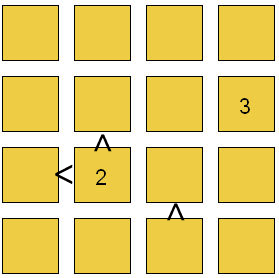# Childrens Hutosiki Puzzles

Hutosiki is a fun puzzle for children. It is quite quick to solve once you get the idea, but is a good test of logic and awareness of mathematical relationships and the ability to understand whether one number is greater than or less than another.

You must complete the 4 x 4 square so that the numbers 1 - 4 are present once in each row and column. You can work out where each number must go by observing the rule above combined with the aid of the greater than ( > ) and less than ( < ) signs that appear in the puzzle. Thus if we see that '2 > x' we know that x must be 1. If we see that '2 < x' we know that x must be 3 or 4.Hutosiki Puzzle from Clarity Media.